# Engineering Mechanics - PKRB: Work and Energy

### Exercise :: PKRB: Work and Energy - General Questions

• PKRB: Work and Energy - General Questions
6.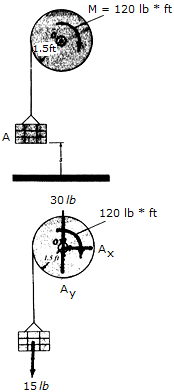A motor supplies a constant torque or twist of M = 120 lb • ft to the drum. If the drum has a weight of 30 lb and a radius of gyration of k0 = 0.8ft, determine the speed of the 15-lb carte A after it rises s = 4 ft starting from rest. Neglect the weight of the cord.

 A. v = 49.1 ft/s B. v = 29.6 ft/s C. v = 26.7 ft/s D. v = 44.3 ft/s

Explanation:

No answer description available for this question. Let us discuss.

7.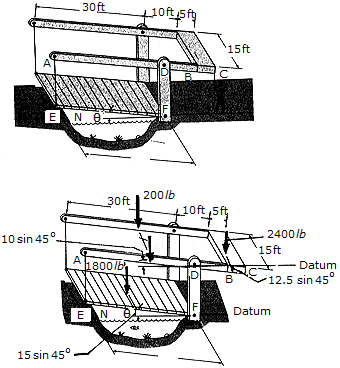The small bridge consists of an 1,800-lb uniform deck EF (thin plate), two overhead beams AB (slender rods), each having a weight of 200 lb, and a 2,400-lb counterweight BC, which can be considered as a thin plate having the dimensions shown. The weight of the tie rods AE can be neglected. If the operator lets go of the rope when the bridge is at an at-rest position,= 45°, determine the speed at which the end of the deck E hits the roadway step at H,= 0°. The bridge is pin-connected at A, D, E, and F.

 A. vE = 5.91 ft/s B. vE = 8.21 ft/s C. vE = 6.12 ft/s D. vE = 6.36 ft/s

Explanation:

No answer description available for this question. Let us discuss.

8.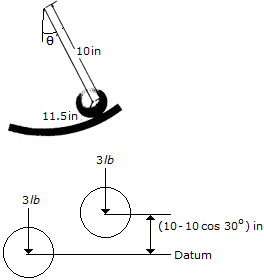If the 3-lb solid sphere is released from rest when= 30°, determine its angular velocity when= 0°, which is the lowest point of the curved path having a radius of 11.5 in. The sphere does not slip as it rolls.

 A.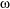= 15.17 rad/s B.= 18.13 rad/s C.= 21.5 rad/s D.= 17.15 rad/s

Explanation:

No answer description available for this question. Let us discuss.

9.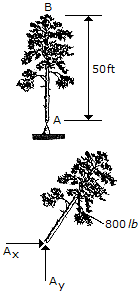An 800-lb tree falls from the vertical position such that it pivots about its cut section at A. If the tree can be considered as a uniform rod, pin-supported at A, determine the speed of its top branch just before it strikes the ground.

 A. vB = 69.5 ft/s B. vB = 80.2 ft/s C. vB = 139.0 ft/s D. vB = 56.7 ft/s

Explanation:

No answer description available for this question. Let us discuss.

10.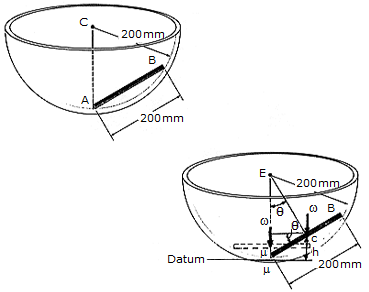THe 500-g rod AB rests along the smooth inner surface of a hemispherical bowl. If the rod is released from the position shown, determine its angular velocityat the instant it swings downward and becomes horizontal.

 A.AB = 11.70 rad/s B.AB = 3.04 rad/s C.AB = 3.90 rad/s D.AB = 3.70 rad/s